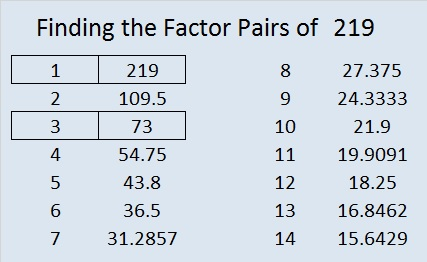# 219 and Level 1

• 219 is a composite number.
• Prime factorization: 219 = 3 x 73
• The exponents in the prime factorization are 1 and 1. Adding one to each and multiplying we get (1 + 1) x (1 + 1) = 2 x 2 = 4. Therefore 219 has 4 factors.
• Factors of 219: 1, 3, 73, 219
• Factor pairs: 219 = 1 x 219 or 3 x 73
• 219 has no square factors so its square root cannot be simplified. √219 ≈ 14.799Excel file of puzzles and previous week’s factor solutions: 10 Factors 2014-09-011.Paula
•ivasallay